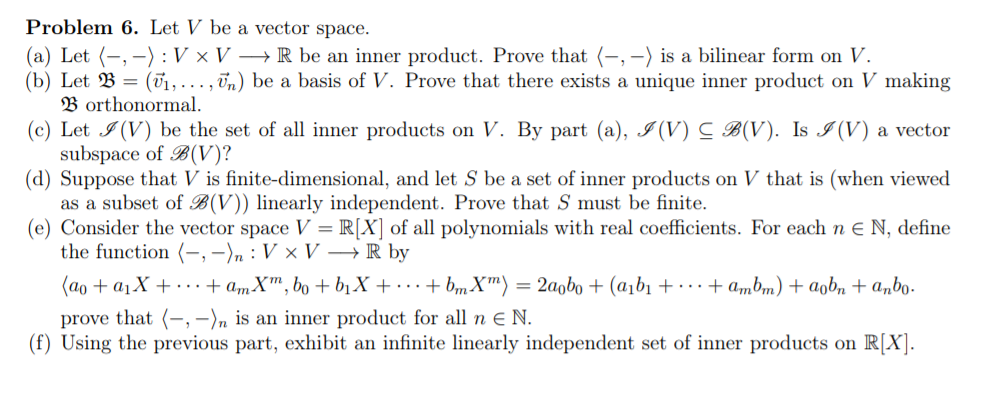# Solved: Problem 6. Let V Be A Vector Space (a) Let (--) : V X V --> R Be An Inner Product. Prove That (-, -) Is A Bilinear Form On V. (b) Let B = (1, ... ,T,) Be A Basis Of V. Prove That There Exists

By |Problem 6. Let V be a vector space (a) Let (--) : V x V --> R be an inner product. Prove that (-, -) is a bilinear form on V. (b) Let B = (1, ... ,T,) be a basis of V. Prove that there exists a unique inner product on V making Borthonormal. (c) Let (V) be the set of all inner products on V. By part (a), J(V) C B(V). Is J(V) a vector subspace of B(V)? (d) Suppose that V is finite-dimensional, and let S be a set of inner products on V that is (when viewed as a subset of B(V)) linearly independent. Prove that S must be finite. (e) Consider the vector space V = R[X] of all polynomials with real coefficients. For each n E N, define the function -, -)n : V x V > R by (?? + aj? + ... +amX", bo + biX +:..+ bm X") 3D 2?gbo + (ajbi +... +ambm) + agb, +anbo- prove that (-, -)n is an inner product for all n E N. (f) Using the previous part, exhibit an infinite linearly independent set of inner products on R[X].